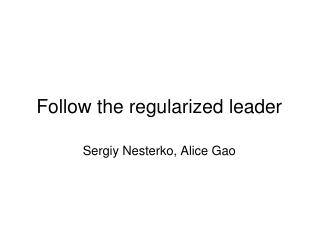DownloadDownload PresentationTélécharger la présentation- - - - - - - - - - - - - - - - - - - - - - - - - - - E N D - - - - - - - - - - - - - - - - - - - - - - - - - - -
##### Presentation Transcript

3. Introduction - problem • Online decision/prediction • Each period, need to pick an expert and follow his "advice" • Incur cost that is associated with the expert we have picked • The goal is to devise a strategy to incur a total cost not much larger than the minimum total cost of any expert

4. Online decision problems • Shortest paths • Tree update problem • Spam prediction • Potfolio selection • Adaptive Huffman coding • etc

5. Why not pick the best performing expert every time? • Suppose there are two experts, and cost sequence of (0,1), (1,0), (0,1), ... • Picking a leader every time would give the cost of t at time t, whereas the best expert would have incurred a cost of about t/2 • Aggravated if there are more experts, prone to adversarial action

6. Instead, follow perturbed leader • The main topic of the first paper we are considering today • Different from the weighted majority by the way randomness is introduced • Applies to a broader set of problems (for example, tree update problem) • Is arguably more elegant • However, the idea is the same: give more chance for the leader(s) to be selected, and be random in your choice

7. The algorithm, intuitive version • At time t, for each expert i, pick p_t[i] ~ Expo(e) • Choose expert with minimal c_t[i] - p_t[i] c_t[i] is the total cost of expert i so far

8. Example: online shortest path problem • Choose a path from vertex a to vertex b on a graph that minimizes travel time • Every time, have to pick a path from a to b, which is when we learn how much time is spent on each edge • Online version: treat all possible paths as experts

9. Online shortest path algorithm • Assign travel time 0 to all edges initially • At every time t and for every edge j, generate an Expo p_t[j], assign every edge weight of c_t[j] - p_t[j], where c_t[j] is the total time on edge j so far • Pick a path with smallest total aggregate travel time

10. The experts problem - why following the perturbed leader works • Can assume that the only p[i] is generated for every expert for all periods to build intuition • if so, expert i is a leader if p[i] > v, for some v, dependent on all other experts' costs and perturbations • Expert i stays a winner, if p[i] > v + c[i] • Then can bound the probability that i stays the leader:

11. Follow the regularized leader (1/2) • Similar to the follow-the-perturbed-leader algorithm • Instead of adding randomized perturbation, add a regularizer function in order to stabilize the decision made, and thus leading to low regret • Choose a decision vector that will minimize cumulative cost + regularization term  • Regret bound:  Average regret -> 0 as T -> +infinity

12. Follow the regularized leader (2/2) • Main idea for proving regret bound: • The hypothetical Be-The-Leader algorithm has no regret.  If FTRL chooses the decisions to be close to BTL, then FTRL would have low regret.  • Tradeoff for choosing a regularizer • If range of the regularizer is too small, cannot achieve sufficient stability. • If range of the regularizer is too large, we are too far away from choosing the optimal decision.

13. Weighted majority • Can be interpreted as a FTRL algorithm with the following regularizer. • Update rule:

14. Gradient descent • Can be interpreted as a FTRL algorithm with the following regularizer:  • Update rule:

15. Online convex optimization • At iteration t, the decision maker chooses x_t in a convex set K. • A convex cost function f_t: K -> R is revealed, and the player incurs the cost f_t(x_t). • The regret of algorithm A at time T is • total cost incurred - cost of best single decision • Goal:  Have a regret sublinear in T, i.e. in terms of average per-period regret, the algorithm performs as well as the best single decision in hindsight. • Examples: the experts problem, online shortest paths

16. Online convex optimization • The follow the regularized leader algorithm  • The primal-dual approach

17. The primal-dual approach • Performing updates and optimization in the dual space defined by the regularizer • Project the dual solution y_t into the solution x_t in the primal space x_t using Bregman divergence • For linear cost functions, the primal-dual approach is equivalent to the FTRL algorithm.

18. Discussion • Would you be able to think of a way to connect FTRL algorithms (e.g. weighted majority) to market scoring rules? • The algorithms strive to achieve single best expert's performance, what if it is not very good? • Tradeoff between speed of execution/performance of experts for a given problem would be interesting to explore